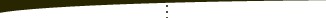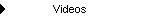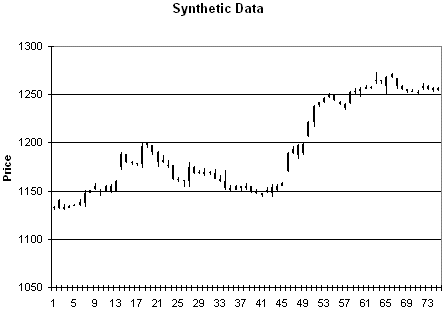About   Products   Support   Videos   Purchase   ContactThe Breakout Bulletin The following article was originally published in the December 2005 issue of The Breakout Bulletin.   Create Your Own Price Data: Creating and Using Synthetic Price Data One of the problems often encountered when developing and testing trading systems is insufficient data to produce reliable test results. As I've discussed before in this newsletter, system testing results are more reliable with more data. Generally speaking, the more data we can test on, the better. In his book "Beyond Technical Analysis, 2nd Ed.," Tushar Chande addresses this problem by demonstrating how to develop synthetic price data using a method he calls "data scrambling" [reference 1].   His basic idea is to start with an actual price series and randomly sample from it to create a "synthetic" price series. However, rather than sampling the prices directly, we sample from the price changes. Consider the spreadsheet shown below in Fig. 1.Figure 1. Spreadsheet for creating synthetic price data. Original price data (A - F) is converted to price changes (H - K).   The first six columns contain the original price data. Columns H - K convert the price data into price changes by subtracting the close of the previous bar. For example, the open becomes the open minus the previous close. The high becomes the high minus the previous close, and so on. It's necessary to work with price changes rather than absolute prices in order to preserve the price changes from bar to bar.   After calculating all the price changes, the next step is to randomize the order of the price changes. This is a little different from the method proposed by Chande who describes using random sampling with replacement. In sampling with replacement, you would randomly select rows from the price change columns and use those to construct the new prices. In sampling with replacement, you might select the same row more than once. Randomizing the order of the price changes is random sampling without replacement. This means we randomly sample from the price changes, but we select each row exactly once.   To do this in the spreadsheet, we copy the columns containing the price changes (H - K) and paste the values into new columns (N - Q); see Fig. 2 below. The important point is that we paste the values only -- not the formulas. We then add an additional column (M) to the left of the copied columns to contain random numbers.Figure 2. Continuation of spreadsheet from Fig. 1. Price changes (H - K) are copied (values only) to columns N - Q and used to create synthetic prices (S - V).   Random numbers can be produced in Excel using the Rand() function. Now that we have the price changes and the column of random numbers, we select the four columns of price changes plus the column of random numbers (M - Q) and sort the columns (Sort command, Data menu) by the column of random numbers (M). This will randomize the order of the price changes. Notice that we sort the columns of price changes together, so the data for each bar stay together.   Finally, the synthetic prices are generated by adding the price changes back to the closing prices. For example, the Open - Close price change is added to the prior close to create the synthetic open price. We start with a reference closing price, which is used for the first row. In Fig. 2, the reference closing price is 1131.75, which appears by itself at the top of the "Synthetic Prices" section in column V. Subsequent rows are created by adding the price changes in that row to the closing price for the prior row. In this way, each price bar is relative to the previous bar's close.   As an example of the type of price data this produces, consider Figs. 3 - 6. Fig. 3 is price plot of the original data. Figs. 4 - 6 contain three different sets of synthetic prices generated by randomizing the price changes of the original data as described above.Figure 3. Original price data.Figure 4. Synthetic prices generated from the data in Fig. 3.Figure 5. Synthetic prices generated from the data in Fig. 3.Figure 6. Synthetic prices generated from the data in Fig. 3.   The spreadsheet that performs these calculations is located on the Free Downloads page on my web site. To use the spreadsheet, follow the instructions in the comment near the top of the spreadsheet in cell D1, which contains "Read ->". Basically, all you need to do is copy your own price data over the data in the spreadsheet, then copy the price change columns to the columns labeled "Price Changes -- Copied Values." Make sure to copy the values only. Fill down as needed or delete rows if your data require more or fewer rows than the data already in the spreadsheet. Then select the copied price change columns and the column of random numbers (M - Q) and sort everything by the column of random numbers (M). To recreate a valid file or price data, copy the synthetic prices (S - V) back into your original file of data next the date/time columns. This will produce a file of synthetic prices with valid dates/times.   A few points about this approach are worth discussing. First, this method preserves not only the price changes but the price relationships within each bar. It's basically randomizing the order of the bars but keeping each bar the same. The only price characteristic that's being altered is the ordering of the bars. The advantage of this approach is that it preserves quite a lot of information about the price series while generating different price formations in the new, synthetic series. However, if you have a trading system that's based on price patterns or methods that depend on a specific ordering of the price bars, there's no reason to think your system will hold up when tested on the synthetic data. For example, if you've found that four up closes in a row is a valid buy signal, you probably shouldn't expect to find that result in a synthetic price series. If that particular price pattern is useful, it's probably because it captures some nonrandom market tendency, such as a psychological response to a strong, short-term trend. If you randomize the price changes, you'll lose that pattern in the synthetic price series.   Also, while the synthetic price series may look much different than the original price series, it still contains the same statistical distribution of price changes. Over time, markets change. One characteristic that can change is the distribution of price changes. Synthetic data as described here won't address that.   Applications of Synthetic Price Data Keeping in mind the points just made, there are two applications of synthetic price data that I'll discuss. The first application is the one recommended by Chande [reference 1]; namely, using synthetic data for system testing. Chande describes this use as true out-of-sample testing. Certainly, a system that performs well on synthetic price data is more robust than one that only does well on historical data. It implies that the system is insensitive to the kind of price-change randomization used to generate the synthetic data. Generally speaking, the more market characteristics that a system is insensitive to, the better. For example, if I had a system that was insensitive to price volatility, it would be better than a system with similar performance that could break down with changes in volatility. A system that holds up well when the price changes are re-ordered, as with a synthetic price series, is better than a system that breaks down on synthetic data. However, there's no guarantee that a system that performs well on synthetic data will hold up as well in the future. As noted above, the distribution of price changes could drift over time or even change suddenly, and synthetic prices can't test for this.   Provided you're testing a system that should in principle hold up on synthetic data, I would suggest an extension of Chande's approach. Instead of just testing a system on synthetic data, why not create a very long synthetic price series and optimize the system's parameter values on the synthetic price series? How long a series is necessary? As noted in previous newsletters, the more trades used in the optimization, the better. If you can create a synthetic price series that generates 500 trades, that would be better for optimization than one with only 100 trades. After optimization, test the system with the optimized parameter values "out-of-sample" on the actual price data. To provide even better out-of-sample testing, divide the original historical data into two segments. Use the first segment to create the long, synthetic price series for optimization. Save the second segment for true out-of-sample testing of the optimized system. This has the following advantages:   You're optimizing over a long price history that generates a large number of trades. The price data include random patterns so the resulting parameter values will not be fit to a small number of historical price patterns that may never repeat. By saving the actual historical data for out-of-sample testing, your final test results will give you confidence that the system is capable of performing well on "real" data.   In principle, one of the advantages of synthetic prices is that you can create arbitrarily long series. To do this in practice using the spreadsheet described above can be a bit tricky, however. Here's one way to do it. Copy the data in the price change columns (N - Q) and paste the data directly below the existing numbers in the same columns, which will double the length of the columns. Repeat the process as many times as necessary to get the length you want. Then fill-down the random number column (M) and the synthetic price columns (S - V). Now sort the price change columns (N - Q) by the random number column (M). This will randomize the price changes and produce the synthetic prices in columns S - V. The only problem is that you'll need to assign dates/times to the prices in order to create your finished price file. If the data are daily bars, so that each bar has a different, consecutive date, this is relatively easy. Convert the dates to numbers by formatting the date column as "text." Then increment each date value, which is now a number, by 1, starting at the last row for which you have date information available. For example, if the last row for which you have dates is row 546 and the date is 11/21/2005, then you would convert this date to a number (in this case, it's 38677) by formatting the cell as text. Then you set the date cell for the next row to A546 + 1 (assuming dates are in column A). The date for the next row would be A547 + 1, etc. You can fill down this formula to increment the date by 1 in each successive row. Then reformat the cells as "dates." Intraday data may require a little more work because several rows of data will have the same date, but the idea is the same.   The second application for synthetic price data that I want to discuss is testing price patterns. Above, I suggested that if your trading system is based on a price pattern that relies on a specific pattern of relative prices, then you'll probably lose that pattern when you randomize the price changes to create the synthetic price data. In this case, our goal is not to come up with a system that is insensitive to price change patterns, as it would be if we were using synthetic data to optimize our system. Quite the opposite. We've found a price pattern that we believe represents some sort of nonrandom market phenomenon, and our goal is to determine if the pattern has any validity.   Let's say, for example, that we've found a pattern that seems to predict that the market will be higher five days later with 60% accuracy. We need to know if 60% accuracy is meaningful or if we could get that kind of accuracy just by random chance. If random chance can produce that kind of accuracy, then our price pattern is not meaningful. This is where the synthetic price data come in. Because the synthetic price data are generated by randomizing the price changes, we can search for our pattern on the synthetic data to determine the accuracy of the pattern on random prices. In order for our price pattern to be meaningful and not just a byproduct of random chance, the pattern should have a higher accuracy on the real data than on most (in a statistical sense) of the synthetic price data.   As an example, we'll consider a pattern for the E-mini S&P 500 futures on 135 min bars over the date range 2/13/2002 to 11/21/2005. 135 min bars divide the day session evenly into three parts (think morning, mid-day, and afternoon session).   The price pattern is as follows: Lowest low of the last 20 bars is found within the past 5 bars High - low range less than the average high - low range over the past three bars Close above previous close Close above average close over past 180 bars   We'll evaluate this pattern by calculating the probability that the market is higher five days later.   Results On Original Data:     65.4% of time (34 of 52 patterns), close was higher 5 days later.   On Synthetic Data:        Series        Probability of higher close 5 days later       1                 55.9%        2                50.0        3                50.0        4                40.8        5                49.1        6                49.1        7                50.0        8                50.0        9                42.9        10               40.8   Each series consisted of a different synthetic price series created from the original data. Over all 10 series, the average probability is 47.86 +/- 4.83%. In other words,  averaging those 10 probability results, the average accuracy of the 10 series is 47.86% and the standard deviation is 4.83%. Ideally, we'd like to have about 30 series to ensure that the distribution is statistically valid, but for the sake of illustration, we'll assume these 10 are sufficient.   In general, 99.9% of normally distributed data are less than three standard deviations above the mean. In this case, three standard deviations above the mean is 47.86 + 3 * 4.83 or 62.4%. In other words, 99.9% of the results from the synthetic price series will be less than 62.4%. Since our accuracy on the actual data was 65.4%, the accuracy of our price pattern is greater than 99.9% of the accuracy values calculated from the synthetic data, which implies that the accuracy is not due to random chance but due to some other phenomenon. This should give us some reassurance that the price pattern is capturing something other than just random noise.   The two uses of synthetic price data described here for evaluating trading systems are quite different from one another. In one case, we're testing or optimizing our trading system on the synthetic prices with the expectation that our system will perform well even on the randomly generated synthetic data. In the other case, we expect that our price pattern will only work well on the original data. Clearly, the way we use synthetic data of the type described here depends on the kind of system we're analyzing. Provided you keep this fact in mind, synthetic price data can be a useful tool to help develop and analyze your trading systems.   Reference 1. Tushar Chande, Beyond Technical Analysis, 2nd ed., John Wiley & Sons, Inc., New York, 2001, pp. 346-352.     That's all for now. Good luck with your trading.   Mike Bryant Breakout FuturesCopyright © 2001-2013 Breakout Futures Copyright © 2013-2017 Adaptrade Software